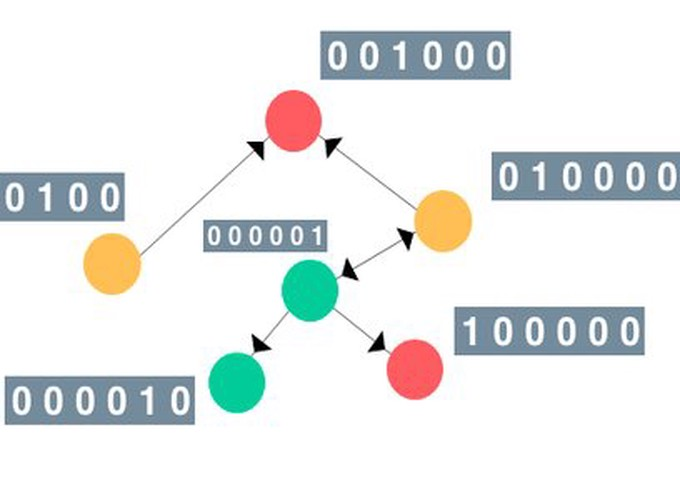Image credit: Medium

# Graph Convolution Network Classifier with Sparse Adjacency Matrix Prior

Please click on the ‘Code’ button displayed above to view project source code in GitHub.

## Abstract

Benchmarking performances of Fully Connected Network (FCN), Deep Convolutional Neural Network (CNN) & Graph Convolution Network with 3 different priors on MNIST.

## Available Models

``````1. Fully Connected
2. Graph (with my Custom Adj Matrix - default)
3. Convolution
4. Graph (with Gaussian Adj Matrix)
5. Graph (with Trainable Adj Matrix)
``````

## Implementation of a Graph Neural Network Classifier with 3 different priors

``````1. Sparse Adjacency Matrix (Feng et al., 2020)
2. Gaussian Adjacency Matrix & Normalization as per (Kipf & Welling et al., ICLR 2017)
3. Trainable Adjacency Matrix (Predict Edges)
``````

## Requirements

``````1. Python 3
2. PyTorch
3. Numpy
4. Networkx
5. Scipy
``````

## Usage

``````1. python graph_neural_network.py --model fc
2. python graph_neural_network.py --model conv
3. python graph_neural_network.py --model gaussian_graph
4. python graph_neural_network.py --model graph --pred_edge
5. python graph_neural_network.py --model graph
``````